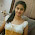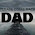## Fighting With PHP operators:

In all programming languages, operators play a key role in performing tasks with variables and values. You might have knowledge about "." or assignment operator"=".

There are many operators in PHP. Here these are categorized as :

1. Assignment Operators
2. Arithmetic Operators
3. Comparison Operators
4. String Operators
5. Increment / Decrement Operators
Here, i will shed light on each of them with a code example. so let us go

## Assignment Operators:

These operators are used to assign some value to some variable or to set a variable to some other variable value. Such operations are completed with assignment operator denoted by "=" equal sign. For example

\$myName  = 'Code2Learn'; // Assign a value to some variable
\$myBlog    = \$myName;      // assign a variable value to another variable

Now both them have the same value, a string ''Code2Learn".

## Arithmetic Operators:

I am practicing math everyday, is that right? yes then i must have knowledge about Arithmetic operations (Subtraction, Addition , Multiplication, Division), To perform all these actions using PHP, we have to use specific operators assigned for each operations. (+,-,*,/)

Let us do this with an example

\$variable1 = 6; \$variable2 = 2;
\$sum        = \$variable1+\$variable2; // This will Add two variables and result8 \$sub = \$variable1-\$variable2;         // This will Subtract two variables and
result 4
\$mul = \$variable1*\$variable2; // This will Multiply two variables and result 12 \$div = \$variable1/\$variable2;  // This will Divide two variables and result 3

## Comparison Operators:

Comparison operators allows you to compare values of variables. For example if i have to check two values, whether A equals B or not, I will use comparison operator to compare.

I have to check \$a=4 and \$b=5 then result will show that these are not equal.
i.e

\$a = 4;
\$b = 5;
if(\$a==\$b)
{
echo 'Both are equal';
}
else
{
echo 'Different Values';
}

Here i would like to mention one important thing in Comparison operators and that is difference between "==" and "===" and the difference is that first comparison operator only compare two variables and it does not check their type(String or Integer), on the other hand second operator compare variable and their type too. If two variable are with same value, but one with string and one with integer, First one operator will return you true and second will return False.

For Example:

2==2 True
5==="5" False

## String Operator:

In previous lines i have let you know how to add two values, but what if i have to add two strings? In PHP, an operator is denoted by "." is used to add two strings. This operation is called concatenation. For Example:

\$start_string   = 'Hello';
\$end_string    = 'World';
\$space           = ' ';
\$final_value = \$start_string.\$space.\$end_string;
echo \$final_value // Output should be Hello World

## Increment/ Decrement Operators:

Increment and Decrements operators are usually used in loops or sometime in conditional statements.

I will straightly go to play with these operators.Example

\$myValue=1;
\$myValue++;
//It will Return myValue and then Increment this. Output 1
++\$anyValue;
//Will increment this and then return MyValue. Output 2
\$myValue--;
//Will return myValue and then subtract 1 from this. Output 2
--\$myValue;

Will subtract 1 and the returns myValue Output 1

If you are done with this, You are ready to develop your first script in PHP.

1.2.